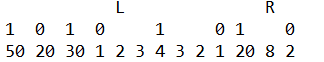# Equality(ISBIAS) Editorial

Author: sahi1422
Tester enchom
Editorialist: Sabre

Prerequisites: STL

Difficulty: Easy

Effective Question: Check if the number of maximal inc/dec contiguous subsequences in a given range(L,R) are equal or not, for Q queries.

L=1,R=13
MIS:3
MDS:4
Verdict: NO

Explanation:

We fix flags(store positions) 0 and 1 to denote the behaviour of an arbitrary subsequence:Going from 0 to 1 implies the subsequence is increasing and vice versa. A single loop running over the length is sufficient for this task.

A few observations follow:

1. This sequence will always be alternating in nature: 010101… or 1010101… and hence any subsequence of these flags will be so too.
2. Not counting the last flag, # of 0’s will give # of MIS and # of 1’s will give the # of MDS in range L=1 and R=N. This is true for any arbitrary subsequence too(with any L or R).

Now we take Q queries each consisting of a “L” and a “R”.

Now each L and R will be between some positions of our preset flags. They may even coincide onto these flags(4 cases). For instance this is one possible case:Now we find the nearest left flag wrt L(is 0 for above) and nearest right flag wrt R(is 0 for above). There maybe 4 possible cases:(00,01,10,11) We treat the cases (00,11) as similiar and (01,10) as similiar.

1. Case: 00/11
Only odd lengths will be allowed.
Eg: 010/101 length=3;
Eg: 01010/10101 length=5;

and so on. So here we note that the #0’s=#1’s if we exclude the last flag. From observation 2 the #MIS=#MDS always for this case.

1. Case: 01/10
Only even lengths will be allowed.
01,0101,010101…,10,1010,101010,…

and so on. So here we note that the #0’s!=#1’s if we exclude the last flag. From observation 2 the #MIS and #MDS will never be equal for this case.

So it all comes down to finding the nearest left flag wrt L and nearest right flag wrt R !

There can be many efficient ways to do this but one way that comes to mind is to use a multiset because of its very efficient insert,find,erase operations.

It will be a simple 5 step process(Assuming L and R are not present initially in the set):

Complexity:
O(N) for fixing the flags. O(logN+logN+logN) for each query, total Q queries so: O(3QlogN).
Overall complexity: O(N+3QlogN) which is O(N+Q).

Sabre

Enjoyed writing my first unofficial editorialThought of writing taster instead of tester(if you know, you know xD)3 Likes

Nice approach. My approach was to store all the increasing and decreasing subsequences as ranges and find out the number of intersections with [L, R]
O(N) to make ranges and O(QLgN) for queries = O(N+QLgN)
My Code

one of the interesting submissions in this problem was this by @avi_gp

1 Like

Thanks, precise code and a good approach too.

1 Like

I did queries in O (1)
Check out my solution
https://www.codechef.com/viewsolution/28757897

3 Likes

I used an auxiliary array initialized it with zeroes than update an index with 1 if it’s greater than previous value in the actual array else remain 0 signifies that whether sequence increased or decreased at that index.

I observe that if the value at L+1 is not equal to the value at R then answer is yes
Else No

2 Likes

https://www.codechef.com/viewsolution/28967994
I got TLE in this solution , please tell how to avoid it?

U r getting tle bcoz u iterating for each given ranges making complexity of your code of an order of O (n^2).
Just check above solutions for an idea.

1 Like

Because its O((R-L)Q) and (R-L) in worst case can be N so O(NQ) which is of order 10^10, you need to reduce this order to 10^5-10^6 ish. Read the editorial and check solutions posted above.

Quite commendable nice…1 Like

Thank you

Please tell me where i am wrong in my approach!

https://www.codechef.com/viewsolution/28901776

that was amazing!

1 Like

How did you got this approach…is it just by observing the pattern in auxiliary array or there is some logic behind it ??

1 Like

Thank you!!

i used segment tree for this however got tle .complexity was just O(Qlog(N)+N).also got segmentation for 1st case.can you take a look at the solution.
https://www.codechef.com/viewsolution/28976764

It is an observation but a true fact
If the values at L+1 and R is same then the no. Of increase and decrease in between will not
Will always diff by at least 1 you can check it out. But if the values are different then for sure the increase and decrease is equal.

I am getting TLE in some of the test cases…can somebody please explain where i am going wrong??
here is my submission :- https://www.codechef.com/viewsolution/28973671

I am not getting the approaches used in above solutions as I am new to programming .
Can you explain how to reduce time complexity of a written code , for example - reducing complexity of : https://www.codechef.com/viewsolution/28967994
Is there any way to reduce complexity?

There is no need to count the number of increasing/decreasing sub sequences. Just observe the nature of sequence(inc/dec) formed by first two and the last two elements of the series between the given L, R bounds ( both inclusive). If the nature of both sequences is same then verdict is NO, otherwise YES. It’s because after the end of every increasing sequence, there has to be a decreasing sequence before the next increasing sequence begins. Therefore in any given L-R span, the difference of counts of both of these sequences cannot be more than 1. Think about it. Here’s my solution : ISBIAS solution

7 Likes

Try increasing the size of segment tree.